September 30, 2023

# Circle| Circle formulas, Parts and Properties

The shape we are most familiar with in our environment is a circle. Numerous items that remind us of a circle are present in daily life. An object’s shape is said to be circular if it resembles a circle. Circular items include the wheel, the coins, the full moon, and many others. A circle is described when a point P moves in a plane such that its distance from a fixed point in the plane remains constant.

Contents

# Circle

A circle is a specific shape that is described as a collection of points in a plane that are all equally spaced from the circle’s centre. Any line that runs through the centre of the circle and joins two points on the boundary of the circle is known as the diameter of the circle. The distance from any point on the circle to its centre is known as its radius. A circle’s radius is equal to half of its diameter. The area of a circle refers to the area that it occupies and the perimeter of a circle is called its circumference.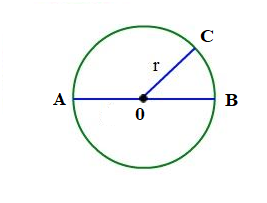Where O is the center of the circle

0C =r denotes the radius of the circle

AB = diameter of the circle

## Parts Of the Circle:

Chord of a circle : The chord of the circle is a line segment whose end points are on the circle. In the given figure AB, EF and CD are chords of a circle with centre O. The  diameter is the longest chord of the circle.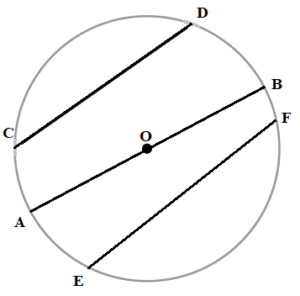Arc of a circle :

A circle’s arc is referred to as a portion or section of its circumference. A semicircular arc is one whose length is exactly half that of a circle. This can be expressed as the letter AB with a curved line above it, such as and read as “arc AB”.

Minor and Major arcs of the two parts of a circle determined by a chord AB , the smaller part is called the minor arc and the greater part is called the major arc of the circle.Segments of a circle:

A chord of a circle divides the circular region into two parts. Each part is called a segment of a circle.  The segment containing the minor arc is called the minor segment and the segment containing the major arc is called the major segment of the circle.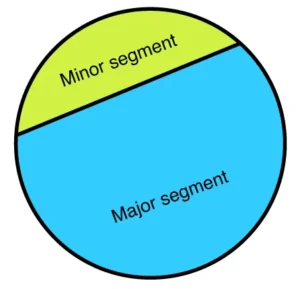Sector of a circle :

A sector is a portion of a circle is covered by two radii and an arc.   A sector of a circle is called a minor sector if the minor arc  of the circle is a part of its boundary . A sector of a circle is called a major sector if the major  arc  of the circle is a part of its boundary .Semicircles have two equally sized sectors, which are separated by diameter.

### Circle Formulas:

• Diameter of the circle = 2 x radius = 2
• Area of circle =
•  Perimeter or Circumference of the circle =
• Semicircle :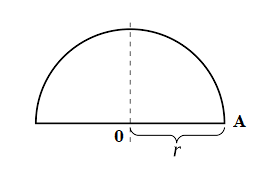(i)Area =                (ii)

• Quadrant  of circle :(i) Area  =                  (ii) Perimeter   =

• Circular ring (Area of annulus) :(i) Area  =              (ii) Outer circumference =          Inner circumference=

• Sector of a circle:(i) If is measured in degrees then area of sector =

(ii) If is measured in  radians then area of sector =

(iii) If length of the arc is given then area =   where l is the length  of the  arc of the sector.

(iv) Perimeter =

Segment of a circle: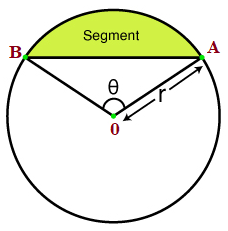(i) If is measured in degrees then area of segment =

(ii) If is measured in  radians then area of  segment  =

(iii) Perimeter =

Equation of a circle: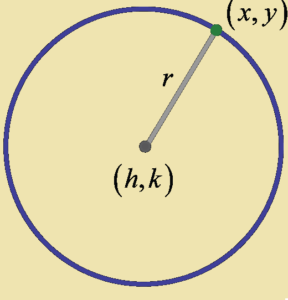•  Standard form of equation of a circle with centre   and radius is
• General equation of a circle  is   with centre and radius

You might also be interested in:#### Bina singh

View all posts by Bina singh →
ssc chsl 2023 tier 1 cut off NIRF Rankings 2023 : Top 10 Engineering colleges in India CBSE Compartment Exam 2023 Application Form SSC CGL 2023 Notification: Important Dates UPSC Recruitment 2023